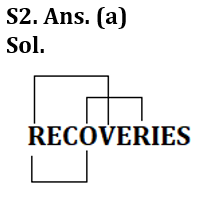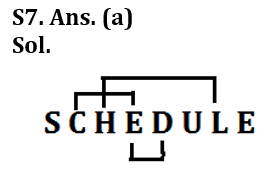Latest Banking jobs   »

# Reasoning Ability Quiz For IDBI AM/ Bank of India PO 2023 -3rd March

Q1. If all the letters in the word ‘MIGRATED’ are arranged in alphabetical order from left to right in such a way that vowels are arranged first followed by consonants, then how many letters are there in between E and T after the arrangement?
(a) two
(b) one
(c) none
(d) three
(e) five

Q2. How many pairs of letters (both forward and backward direction) are there in the word “RECOVERIES” each of which have as many letters between them in the word as they have between them in the English alphabetical series?
(a) Three
(b) One
(c) Two
(d) More than three
(e) None

Q3. If in the number 1839752, 2 is subtracted to each of the digit which is greater than 6 and 1 is added from each of the digit which is less than 6 then how many digits are repeating in the number thus formed?
(a) two
(b) one
(c) none
(d) three
(e) four

Q4. Which of the following elements should come in a place of ‘?’
CE24 GI20 KM16 ?
(a) PR11
(b) OQ12
(c) PQ11
(d) OR12
(e) None of these

Q5. Among A, B, C, D and E, each of them is different weight. D’s weight is more than only one person. B’s weight is more than E and C. A is heavier than E. C is not the lightest person. Who among the following is heaviest person?
(a) B
(b) A
(c) E
(d) Either (a) or (b)
(e) None of these

Q6. If it is possible to make only one meaningful English word from the 1st, 6th, 8th and the 10th letters of the word ‘INFORMATION’, using each letter only once, second letter of that word is your answer. If more than one such word can be formed, your answer is X. If no such word can be formed, your answer is Y.
(a) T
(b) M
(c) O
(d) X
(e) Y

Q7. How many pairs of letters are there in the word ‘SCHEDULE’, each of which have as many letters between then in the word as they have between then in the English alphabet?
(a) Three
(b) One
(c) None
(d) Two
(e) More than three

Q8. In a row total 46 students are seated. Rahul sits 8th from the left end of the row and Riya sits 9th from the right end of the row. If Dinesh sits exactly middle of Rahul and Riya then find the rank of Dinesh from the right end of the row?
(a) 19
(b) 24
(c) 18
(d) None of these
(e) Can’t be determined

Q9. Among A, B, C, D and E, each having scored different marks, B has scored more marks than E and D. B has not scored the highest marks among them. Who among them scored the second-highest marks?
(a) B
(b) C
(c) E
(d) D

Q10. If 1 is subtracted from all the even digits and 1 is added to all the odd digits in the number 3875264, then arrange in ascending order, which of the following digit is third from the right end?
(a) 6
(b) 4
(c) 3
(d) 7
(e) None of these

Q11. The position of how many alphabets will remain unchanged if each of the alphabets in the word ‘SUBSCRIBE’ is arranged in alphabetical order from left to right?
(a) None
(b) One
(c) Two
(d) Three
(e) More than Three

Q12. What will come in place of question-mark (?) in the following series?
AZF BYG CXH DWI ?
(a) ESJ
(b) EUV
(c) EVJ
(d) DST
(e) FRH

Q13. Priya rank is 18th from the top and 6th from the bottom in the class. How many students are there in the class?
(a) 29
(b) 28
(c) 27
(d) 23
(e) None of these

Q14.Among A, B, C, D and E, A is heavier than B but lighter than C. B is heavier than only E. C is not the heaviest. Who among them will be in the middle if they stand in the order of their weight?
(a) A
(b) C
(c) B
(d) D
(e) E

Q15. If 2 is multiply by all the even digits and 1 is Subtracted from all the odd digits in the number 1239475, then arrange all digits in descending order, then which of the following is second from the right end?
(a) 2
(b) 8
(c) 6
(d) 4
(e) 0

Solutions

S1. Ans. (e)
Sol. Original Word-MIGRATED
After operation- AEIDGMRTS3. Ans. (b)
Sol. Original number- 1839752
Obtained number- 2647563

S4. Ans. (b)

S5. Ans. (d)
Sol. B/A > A/B > C > D > E

S6. Ans. (b)
Sol. OMITS8. Ans. (b)
Sol. Rahul is 8th from the left and Riya is 9th from the right.
So, position of Rahul from right is- 46-(8-1) = 39
Thus, number of students between Rahul and Riya is= 29
Dinesh sits exactly between Rahul and Riya, So Dinesh is 24 from right end.

S9. Ans. (e)
S10. Ans. (a)

S11. Ans. (b)
Sol. S U B S C R I B E
B B C E I R S S U

S12. Ans. (c)

S13. Ans. (d)
Sol. Total number of students in the class= 18+6-1= 23

S14. Ans. (a)
Sol. D>C>A>B>E

S15. Ans. (a)
Sol. Original number- 1239475
Obtain Number- 0428864
Descending order- 8864420## FAQs

### What is the selection process of the IDBI AM?

The selection process of IDBI AM is Online exam and Interview.

#### Congratulations!Union Budget 2023-24: Free PDF